Updating search results...

# 35 Results

View
Selected filters:
• CCSS.Math.Content.7.EE.B.3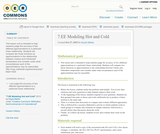Conditional Remix & Share Permitted
CC BY-NC
Rating
4.0 stars

This lesson unit is intended to help students judge the accuracy of two different approximations to a particular linear relationship. Students will compare two linear functions as approximations to the relationship between Celsius and Fahrenheit temperature and consider under what circumstances each of the approximations may be reasonable.

Subject:
Mathematics
Material Type:
Lesson
Author:
Kayla Martin
01/19/2017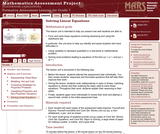Only Sharing Permitted
CC BY-NC-ND
Rating
5.0 stars

This lesson unit is intended to help teachers assess how well students are able to: form and solve linear equations involving factorizing and using the distributive law. In particular, this unit aims to help teachers identify and assist students who have difficulties in: using variables to represent quantities in a real-world or mathematical problem and solving word problems leading to equations of the form px + q = r and p(x + q) = r.

Subject:
Algebra
Material Type:
Assessment
Lesson Plan
Provider:
Shell Center for Mathematical Education
Provider Set:
Mathematics Assessment Project (MAP)
04/26/2013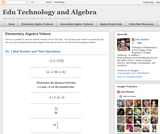Conditional Remix & Share Permitted
CC BY-NC
Rating
0.0 stars

Visually searchable database of Algebra 1 videos. Click on a problem and watch the solution on YouTube. Copy and paste this material into your CMS. Videos accompany the open Elementary Algebra textbook published by Flat World Knowledge.

Subject:
Algebra
Material Type:
Lecture
Lecture Notes
Provider:
Individual Authors
Provider Set:
Individual Authors
Author:
John Redden
04/29/2012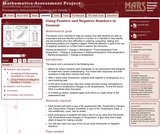Only Sharing Permitted
CC BY-NC-ND
Rating
3.5 stars

This lesson unit is intended to help teachers assess how well students are able to understand and use directed numbers in context. It is intended to help identify and aid students who have difficulties in ordering, comparing, adding, and subtracting positive and negative integers. Particular attention is paid to the use of negative numbers on number lines to explore the structures: starting temperature + change in temperature = final temperature final temperature Đ change in temperature = starting temperature final temperature Đ starting temperature = change in temperature.

Subject:
Numbers and Operations
Material Type:
Assessment
Lesson Plan
Provider:
Shell Center for Mathematical Education
Provider Set:
Mathematics Assessment Project (MAP)
04/26/2013Only Sharing Permitted
CC BY-NC-ND
Rating
4.5 stars

This lesson unit is intended to help assess how well students are able to interpret and use scale drawings to plan a garden layout. This involves using proportional reasoning and metric units.

Subject:
Algebra
Geometry
Ratios and Proportions
Material Type:
Assessment
Lesson Plan
Provider:
Shell Center for Mathematical Education
Provider Set:
Mathematics Assessment Project (MAP)
04/26/2013Conditional Remix & Share Permitted
CC BY-NC
Rating
0.0 stars

Math in Real Life (MiRL) supports the expansion of regional networks to create an environment of innovation in math teaching and learning.  The focus on applied mathematics supports the natural interconnectedness of math to other disciplines while infusing relevance for students.  MiRL supports a limited number of networked math learning communities that focus on developing and testing applied problems in mathematics.  The networks help math teachers refine innovative teaching strategies with the guidance of regional partners and the Oregon Department of Education.

Subject:
Mathematics
Material Type:
Homework/Assignment
Author:
Mark Freed
Tom Thompson
07/27/2020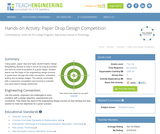Educational Use
Rating
0.0 stars

Using paper, paper clips and tape, student teams design flying/falling devices to stay in the air as long as possible and land as close as possible to a given target. Student teams use the steps of the engineering design process to guide them through the initial conception, evaluation, testing and re-design stages. The activity culminates with a classroom competition and scoring to evaluate how each team's design performed.

Subject:
Engineering
Physics
Material Type:
Activity/Lab
Provider:
TeachEngineering
Provider Set:
TeachEngineering
Author:
Howard Kimmel
John Carpinelli
Ronald Rockland
10/14/2015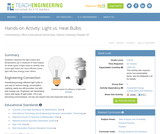Educational Use
Rating
0.0 stars

Students measure the light output and temperature (as a measure of heat output) for three types of light bulbs to identify why some light bulbs are more efficient (more light with less energy) than others.

Subject:
Engineering
Physics
Material Type:
Activity/Lab
Provider:
TeachEngineering
Provider Set:
TeachEngineering
Author:
Jan DeWaters
Susan Powers
09/18/2014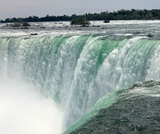Conditional Remix & Share Permitted
CC BY-NC
Rating
0.0 stars

Students first create a diagram that represents the distance a ship drops in each of a series of locks. Students create their diagrams based on a video of an actual ship traveling through the locks. Students need to use contextual clues in order to determine the relative drops in each of the locks.Key ConceptsStudents are expected to use the mathematical skills they have acquired in previous lessons or in previous math courses. The lessons in this unit focus on developing and refining problem-solving skills.Students will:Try a variety of strategies to approaching different types of problems.Devise a problem-solving plan and implement their plan systematically.Become aware that problems can be solved in more than one way.See the value of approaching problems in a systematic manner.Communicate their approaches with precision and articulate why their strategies and solutions are reasonable.Make connections between previous learning and real-world problems.Create efficacy and confidence in solving challenging problems in a real-world setting.Goals and Learning ObjectivesRead and interpret maps, graphs, and diagrams.Solve problems that involve linear measurement.Estimate length.Critique a diagram.

Subject:
Algebra
Material Type:
Lesson Plan
Provider:
Pearson
09/21/2015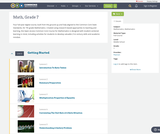Conditional Remix & Share Permitted
CC BY-NC
Rating
0.0 stars

Four full-year digital course, built from the ground up and fully-aligned to the Common Core State Standards, for 7th grade Mathematics. Created using research-based approaches to teaching and learning, the Open Access Common Core Course for Mathematics is designed with student-centered learning in mind, including activities for students to develop valuable 21st century skills and academic mindset.

Subject:
Mathematics
Material Type:
Full Course
Provider:
Pearson
10/06/2016Conditional Remix & Share Permitted
CC BY-NC
Rating
0.0 stars

Students explore the relationship between the flapping frequency, the amplitude, and the cruising speeds of a variety of animals to calculate their Strouhal numbers.Key ConceptsStudents are expected to use the mathematical skills they have acquired in previous lessons or in previous math courses. The lessons in this unit focus on developing and refining problem-solving skills. Students will:Try a variety of strategies to approaching different types of problems.Devise a problem-solving plan and implement their plan systematically.Become aware that problems can be solved in more than one way.See the value of approaching problems in a systematic manner.Communicate their approaches with precision and articulate why their strategies and solutions are reasonable.Make connections between previous learning and real-world problems.Create efficacy and confidence in solving challenging problems in a real-world setting.Goals and Learning ObjectivesAnalyze the relationship between the variables in an equation.Write formulas to show how variables relate.Communicate findings using multiple representations including tables, charts, graphs, and equations.

Subject:
Algebra
Material Type:
Lesson Plan
Provider:
Pearson
09/21/2015Conditional Remix & Share Permitted
CC BY-NC-SA
Rating
0.0 stars

This task was developed by high school and postsecondary mathematics and agriculture sciences educators, and validated by content experts in the Common Core State Standards in mathematics and the National Career Clusters Knowledge & Skills Statements. It was developed with the purpose of demonstrating how the Common Core and CTE Knowledge & Skills Statements can be integrated into classroom learning - and to provide classroom teachers with a truly authentic task for either mathematics or CTE courses.

Subject:
Ratios and Proportions
Material Type:
Activity/Lab
Lesson Plan
Provider:
National Association of State Directors of Career Technical Education Consortium
Provider Set:
Career Technical Education
07/26/2012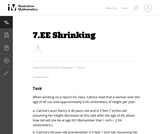Unrestricted Use
CC BY
Rating
0.0 stars

This word problem is based estimating the height of a person over time. Note that there is a significant amount of rounding in the final answer. This is because people almost never report their heights more precisely than the closest half-inch. If we assume that the heights reported in the task stem are rounded to the nearest half-inch, then we should report the heights given in the solution at the same level of precision.

Subject:
Mathematics
Algebra
Material Type:
Activity/Lab
Provider:
Illustrative Mathematics
Provider Set:
Illustrative Mathematics
Author:
Illustrative Mathematics
05/01/2012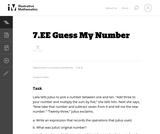Unrestricted Use
CC BY
Rating
0.0 stars

This problem asks the students to represent a sequence of operations using an expression and then to write and solve simple equations. The problem is posed as a game and allows the students to visualize mathematical operations. It would make sense to actually play a similar game in pairs first and then ask the students to record the operations to figure out each other's numbers.

Subject:
Mathematics
Algebra
Material Type:
Activity/Lab
Provider:
Illustrative Mathematics
Provider Set:
Illustrative Mathematics
Author:
Illustrative Mathematics
08/07/2012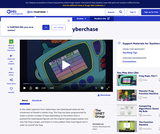Educational Use
Rating
0.0 stars

In this video from Cyberchase, the CyberSquad must figure out the new input/output pattern on Hackerë_í__ larger cyberfrog.

Subject:
Mathematics
Algebra
Material Type:
Lecture
Provider:
PBS LearningMedia
Provider Set:
PBS Learning Media: Multimedia Resources for the Classroom and Professional Development
Author:
U.S. Department of Education
WNET
07/11/2008Rating
0.0 stars

Algebraic Reasoning

Type of Unit: Concept

Prior Knowledge

Students should be able to:

Add, subtract, multiply, and divide rational numbers.
Evaluate expressions for a value of a variable.
Use the distributive property to generate equivalent expressions including combining like terms.
Understand solving an equation or inequality as a process of answering a question: which values from a specified set, if any, make the equation or inequality true?
Write and solve equations of the form x+p=q and px=q for cases in which p, q, and x are non-negative rational numbers.
Understand and graph solutions to inequalities x<c or x>c.
Use equations, tables, and graphs to represent the relationship between two variables.
Relate fractions, decimals, and percents.
Solve percent problems included those involving percent of increase or percent of decrease.

Lesson Flow

This unit covers all of the Common Core State Standards for Expressions and Equations in Grade 7. Students extend what they learned in Grade 6 about evaluating expressions and using properties to write equivalent expressions. They write, evaluate, and simplify expressions that now contain both positive and negative rational numbers. They write algebraic expressions for problem situations and discuss how different equivalent expressions can be used to represent different ways of solving the same problem. They make connections between various forms of rational numbers. Students apply what they learned in Grade 6 about solving equations such as x+2=6 or 3x=12 to solving equations such as 3x+6=12 and 3(x−2)=12. Students solve these equations using formal algebraic methods. The numbers in these equations can now be rational numbers. They use estimation and mental math to estimate solutions. They learn how solving linear inequalities differs from solving linear equations and then they solve and graph linear inequalities such as −3x+4<12. Students use inequalities to solve real-world problems, solving the problem first by arithmetic and then by writing and solving an inequality. They see that the solution of the algebraic inequality may differ from the solution to the problem.

Subject:
Mathematics
Algebra
Material Type:
Unit of Study
Provider:
PearsonRating
0.0 stars

Putting Math to Work

Type of Unit: Problem Solving

Prior Knowledge

Students should be able to:

Solve problems involving all four operations with rational numbers.
Write ratios and rates.
Write and solve proportions.
Solve problems involving scale.
Write and solve equations to represent problem situations.
Create and interpret maps, graphs, and diagrams.
Use multiple representations (i.e., tables, graphs, and equations) to represent problem situations.
Calculate area and volume.
Solve problems involving linear measurement.

Lesson Flow

Students apply and integrate math concepts they have previously learned to solve mathematical and real-world problems using a variety of strategies. Students have opportunities to explore four real-world situations involving problem solving in a variety of contexts, complete a project of their choice, and work through a series of Gallery problems.

First, students utilize their spatial reasoning and visualization skills to find the least number of cubes needed to construct a structure when given the front and side views. Then, students select a project to complete as they work through this unit to refine their problem-solving skills. Students explore the relationship between flapping frequency, amplitude, and cruising speed to calculate the Strouhal number of a variety of flying and swimming animals. After that, students explore the volume of the Great Lakes, applying strategies for solving volume problems and analyzing diagrams. Next, students graphically represent a virtual journey through the locks of the Welland Canal, estimating the amount of drop through each lock and the distance traveled. Students have a day in class to work on their projects with their group.

Then, students have two days to explore Gallery problems of their choosing. Finally, students present their projects to the class.

Subject:
Mathematics
Material Type:
Unit of Study
Provider:
PearsonConditional Remix & Share Permitted
CC BY-NC
Rating
0.0 stars

Students create a bar graph showing the Strouhal numbers for a variety of birds and bats and use their graph and other data to compare the Strouhal numbers of the different animals to analyze variation and to make predictions.Key ConceptsStudents are expected to use the mathematical skills they have acquired in previous lessons or in previous math courses. The lessons in this unit focus on developing and refining problem-solving skills. Students will:Try a variety of strategies to approaching different types of problems.Devise a problem-solving plan and implement their plan systematically.Become aware that problems can be solved in more than one way.See the value of approaching problems in a systematic manner.Communicate their approaches with precision and articulate why their strategies and solutions are reasonable.Make connections between previous learning and real-world problems.Create efficacy and confidence in solving challenging problems in a real-world setting.Goals and Learning ObjectivesAnalyze the relationship among the variables in an equation.Write formulas to show how variables relate.Calculate ranges of Strouhal numbers and use these ranges to make predictions.Communicate findings using multiple representations including tables, charts, graphs, and equations.Create bar graphs.

Subject:
Numbers and Operations
Material Type:
Lesson Plan
Provider:
Pearson
09/21/2015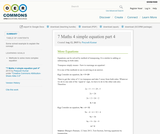Conditional Remix & Share Permitted
CC BY-SA
Rating
0.0 stars

Some solved example to explain the concept

Subject:
Mathematics
Material Type:
Author:
Peeyush Kumar
01/28/2016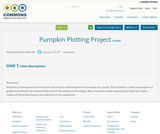Conditional Remix & Share Permitted
CC BY-NC
Rating
0.0 stars

Students create equations that have solutions to ordered pairs of an image on a graph. First students create an image on a graph and identify the ordered pairs for all the points of the image. Next, students create equations so that the x and y values of the ordered pairs are solutions to the equations.

Subject:
Education
Mathematics
Material Type:
Activity/Lab
Homework/Assignment
Author:
Rebecca Layton